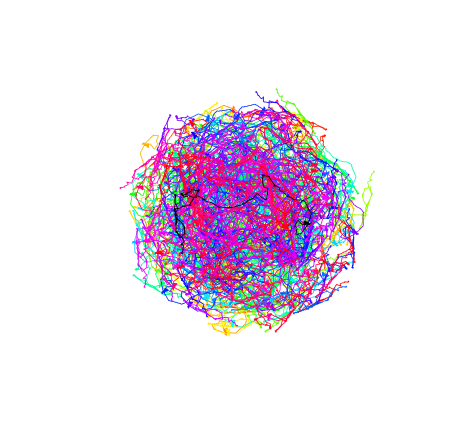# Landscape resistance from spatial randomization

I was intrigued by the idea of running simulations to define landscape resistance as presented in Cushman et al. (2010). Their basic strategy is to use measured track data as a proxy for landscape resistance. They randomly shift and rotate their measured tracks in order to generate support for their paramaterization of landscape resistance by comparing original and transformed tracks. The R code below takes an approximation of their example track and puts it through the same random shifting/rotating procedure.library(raster)
library(sp)
library(maptools)
library(grDevices)
library(XML)

path.mat<-eval(parse(text=gsub('\r\n','\n',xpathApply(htmlTreeParse('http://pastebin.com/HN1u8fpW',useInternal=T),'//textarea',xmlValue))))
names(path.mat)<-c("x","y")
path.mat<-path.mat/10000

path<-Lines(list(Line(path.mat)),ID="p")
pathSL<-SpatialLines(list(path))

rot.list<-list()
for(i in 1:199){
#randomly shift nodes between 0 and 30km
shiftx<-sample(seq(-3,3,0.01),1,replace=T)
shifty<-sample(seq(-3,3,0.01),1,replace=T)
npath.mat<-cbind(path.mat[,1]+shiftx,path.mat[,2]+shifty)
npath<-Lines(list(Line(npath.mat)),ID="np")
npathSL<-SpatialLines(list(npath))

#randomly rotate path
npath.rot<-elide(npathSL,rotate=sample(seq(0,360,0.01),1,replace=T),center=apply(bbox(pathSL),1,mean))
npath.rot.mat<-coordinates(npath.rot)[][]
rot.list[[i]]<-npath.rot<-Lines(list(Line(npath.rot.mat)),ID=paste("npr",i,sep=""))
}
rot.list\$original<-path
all.lines<-SpatialLines(rot.list)

#generate simulated resistance surface
textent<-bbox(all.lines)
nrows<-(textent[1,2]-textent[1,1])
ncols<-(textent[2,2]-textent[2,1])
rsurf<-raster(matrix(NA,nrow=nrows,ncol=ncols))
extent(rsurf)<-textent
rsurf[]<-runif(nrows*ncols)
rsurf<-rsurf>0.8

plot(rsurf)
plot(all.lines,col=c(rainbow(199),"black"))


###Reference > Cushman, S. A., Chase, M., & Griffin, C. (2010). Mapping landscape resistance to identify corridors and barriers for elephant movement in southern Africa. In Spatial complexity, informatics, and wildlife conservation (pp. 349-367). Springer Japan. >